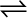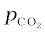7.20 One of the reaction that takes place in producing steel from iron ore is the reduction of iron(II) oxide by carbon monoxide to give iron metal and CO2.

FeO (s) + CO (g)Fe (s) + CO2 (g); Kp = 0.265 atm at 1050K

What are the equilibrium partial pressures of CO and CO2 at 1050 K if the initial partial pressures are: pCO= 1.4 atm and= 0.80 atm?

For the given reaction,
It is given that ${K}_{p}$ =0.265.
Since, ${Q}_{p}>{K}_{p}$, the reaction will proceed in the backward direction.
Therefore, we can say that the pressure of CO will increase while the pressure of $C{O}_{2}$  will decrease.
Now, let the increase in pressure of CO = decrease in pressure of $C{O}_{2}$ be p.
Then, we can write,
Therefore, equilibrium partial of
And, equilibrium partial pressure of